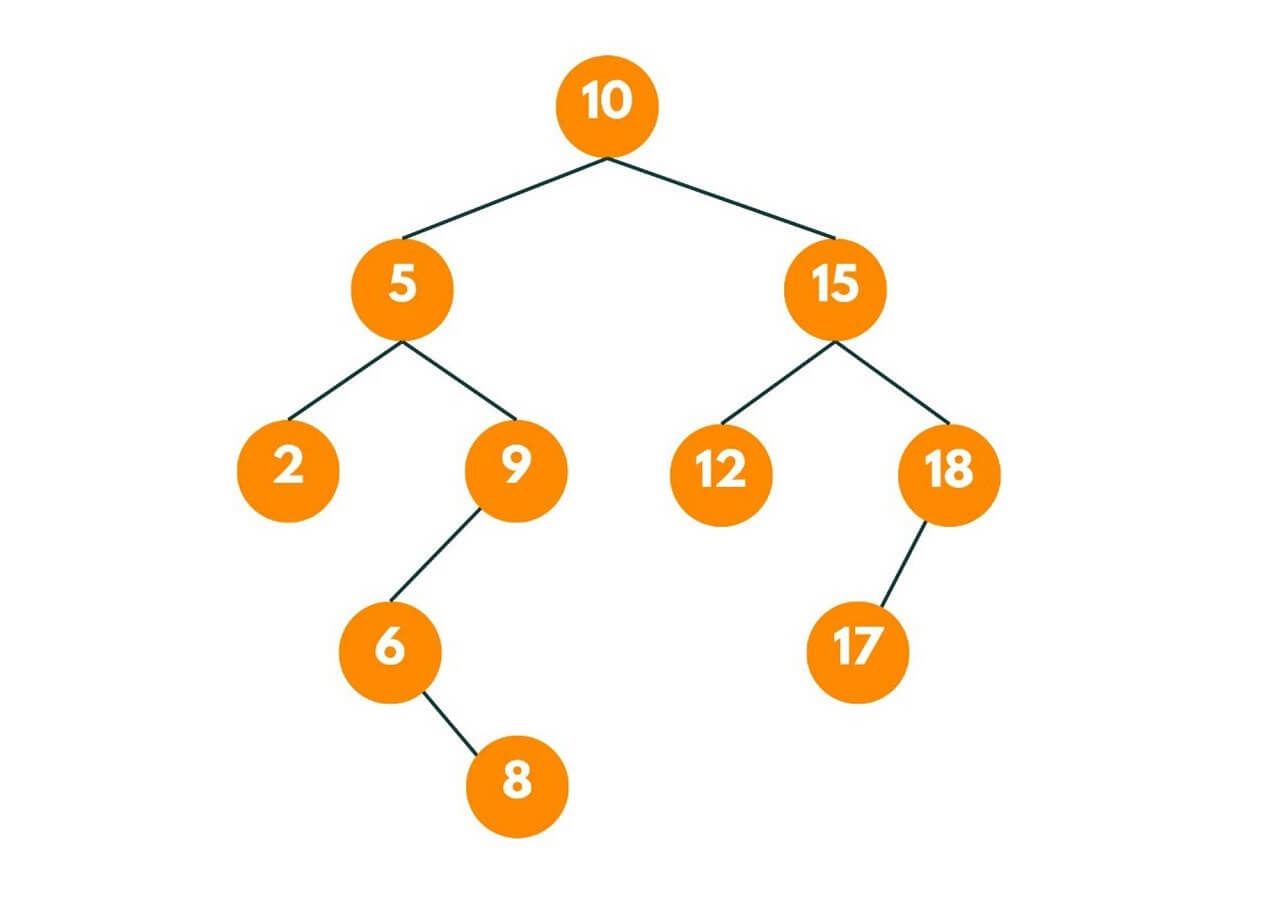# How to Merge Binary Search Trees? (with code)

Binary Search Trees are powerful data structures used to efficiently store and retrieve data in a sorted manner. Sometimes, there arises a need to merge two BSTs into a single tree while preserving the sorted order. This article explores the basics of merging two BSTs and provides an overview of the approach to accomplish this task.

## How to Merge Two Binary Search Trees?

First, let's revise what are binary search trees.

A Binary Search Tree, or a BST, is a hierarchical data structure where each node has two children at most, but the left child contains a smaller key value, while the right child node contains a larger key value. This recursive property holds true for all nodes in the tree. This property allows for efficient search, insertion, and deletion operations.

The following diagram is an example of a Binary Search Tree:Binary Search Trees are particularly useful for searching for a specific key in the tree, you can follow a recursive approach, traversing the tree from the root and moving left or right based on whether the key you are looking for is smaller or larger than the current node's key. There are also variations of binary search trees, such as AVL trees and red-black trees.

Learning how to merge two Binary Search Trees can be useful when working with large databases from different sources. It is also important for Data Analysts, who can combine and analyze multiple datasets for finding common elements and identifying trends.

Now, it's time to solve the problem. But before that, we need the Problem Statement: "Given two binary search trees, print the elements of both BSTs in sorted form."

### Merging 2 BSTs using Stacks

Stacks are very useful when we need to merge two binary search trees using an iterative approach. This approach utilizes the properties of BSTs and the concept of stack to compare and merge the nodes of two BSTs efficiently, without requiring additional space proportional to the size of the trees.

Here's a step-by-step algorithm to achieve this:

1. Create two empty stacks, stack1, and stack2, to store the nodes of the BSTs.
2. Iterate until tree1 is not NULL or tree2 is not NULL or stack1 is not empty or stack2 is not empty:
1. Push all the left nodes of tree1 into stack1 until reaching a NULL node.
2. Push all the left nodes of tree2 into stack2 until reaching a NULL node.
3. If stack1 is not empty and stack2 is empty, pop a node from stack1, add it to the result array, and move it to its right child.
4. If stack2 is not empty and stack1 is empty, pop a node from stack2, add it to the result array, and move it to its right child.
5. If both stack1 and stack2 are not empty, compare the top nodes of both stacks. Pop the smaller node from its respective stack, add it to the result array, and move to its right child.
3. Return the result array containing the merged elements of the BSTs.

Note that the order of steps 2a and 2b can be interchanged, depending on which tree you want to prioritize when the stack for the other tree becomes empty.

Here is the pseudocode to implement it:

```public static int[] getAllElements(TreeNode root1,TreeNode root2) {
stack1 = Stack()
stack2 = Stack()
tree1 = root1
tree2 = root2
int[] result
while(tree1 or tree2 or stack1 or stack2) {
while (tree1) {
stack1.push(tree1)
tree1 = tree1.left
}
while (tree2) {
stack2.push(tree2)
tree2 = tree2.left
}
if (not stack2) or (stack1 and stack1.top().val <= stack2.top().val) {
tree1 = stack1.pop()
result.push(tree1.val)
tree1 = tree1.right
}
else {
tree2 = stack2.pop()
result.push(tree2.val)
tree2 = tree2.right
}
}
return result
}
```

The time complexity of merging two BSTs using this approach is O(m + n), where m and n are the number of nodes in the two BSTs, respectively. The space complexity is O(n) and if the BST is height-balanced BST, then its O(log n).

### Merging Two Binary Search Trees using Tree Traversals

The basic idea is to traverse both trees in an in-order manner, which will give us the elements in sorted order, and then construct a new binary search tree from the sorted elements.

The general approach can be summarized as follows:

1. Perform an inorder traversal on the first BST and store the resulting elements in a list or an array.
2. Perform an in-order traversal on the second BST and append the elements to the existing list or array.
3. Create a new BST using the merged elements from the list or array.
4. Return the root node of the merged BST.

Below is the pseudocode for its implementation:

```function mergeBSTs(BST1, BST2):
mergedElements = []

// Step 1: Inorder traversal of BST1
inorderTraversal(BST1, mergedElements)

// Step 2: Inorder traversal of BST2 and append to mergedElements
inorderTraversal(BST2, mergedElements)

// Step 3: Create a new BST using mergedElements
return constructBST(mergedElements)

function inorderTraversal(node, mergedElements):
if node is null:
return

inorderTraversal(node.left, mergedElements)
mergedElements.append(node.value)
inorderTraversal(node.right, mergedElements)

function constructBST(elements):
if elements is empty:
return null

mid = length(elements) / 2
root = Node(elements[mid])

root.left = constructBST(elements[0:mid])
root.right = constructBST(elements[mid+1:])

return root```

The time complexity of performing an in-order traversal on a BST is O(n), where n is the total number of nodes in the tree. With two trees, we can formulate it O(n1 + n2), where n1 and n2 are the number of nodes in the first and second BST.

The space complexity of this approach is O(n1 + n2), considering the space required for the merged elements and the recursive stack.

## Conclusion

Merging two BSTs can be achieved by performing in-order traversals on both trees and creating a new BST from the merged elements. By understanding the basics, you can now efficiently merge two Binary Search Trees. For more explanation, connect with our DSA tutors online.

### FavTutor - 24x7 Live Coding Help from Expert Tutors!